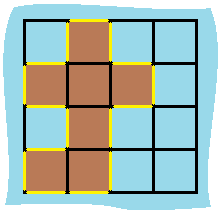##### Welcome to Subscribe On Youtube

Formatted question description: https://leetcode.ca/all/463.html

# 463. Island Perimeter

Easy

## Description

You are given a map in form of a two-dimensional integer grid where 1 represents land and 0 represents water.

Grid cells are connected horizontally/vertically (not diagonally). The grid is completely surrounded by water, and there is exactly one island (i.e., one or more connected land cells).

The island doesn’t have “lakes” (water inside that isn’t connected to the water around the island). One cell is a square with side length 1. The grid is rectangular, width and height don’t exceed 100. Determine the perimeter of the island.

Example:

Input:

[[0,1,0,0],
[1,1,1,0],
[0,1,0,0],
[1,1,0,0]]

Output: 16

Explanation: The perimeter is the 16 yellow stripes in the image below:## Solution

For each cell that is land (the value is 1), check its four sides. For each side, if the side is on the boarder or adjacent to water, then add the perimeter by 1. Finally, return the perimeter.

• class Solution {
public int islandPerimeter(int[][] grid) {
int perimeter = 0;
int rows = grid.length, columns = grid.length;
for (int i = 0; i < rows; i++) {
for (int j = 0; j < columns; j++) {
int cell = grid[i][j];
if (cell == 0)
continue;
if (i == 0 || grid[i - 1][j] == 0)
perimeter++;
if (i == rows - 1 || grid[i + 1][j] == 0)
perimeter++;
if (j == 0 || grid[i][j - 1] == 0)
perimeter++;
if (j == columns - 1 || grid[i][j + 1] == 0)
perimeter++;
}
}
return perimeter;
}
}

############

class Solution {
public int islandPerimeter(int[][] grid) {
int ans = 0;
int m = grid.length;
int n = grid.length;
for (int i = 0; i < m; i++) {
for (int j = 0; j < n; j++) {
if (grid[i][j] == 1) {
ans += 4;
if (i < m - 1 && grid[i + 1][j] == 1) {
ans -= 2;
}
if (j < n - 1 && grid[i][j + 1] == 1) {
ans -= 2;
}
}
}
}
return ans;
}
}

• // OJ: https://leetcode.com/problems/island-perimeter/
// Time: O(MN)
// Space: O(1)
class Solution {
public:
int islandPerimeter(vector<vector<int>>& A) {
int M = A.size(), N = A.size(), ans = 0, dirs = { {0,1},{0,-1},{1,0},{-1,0} };
for (int i = 0; i < M; ++i) {
for (int j = 0; j < N; ++j) {
if (A[i][j] == 0) continue;
for (auto &dir : dirs) {
int x = i + dir, y = j + dir;
ans += x < 0 || x >= M || y < 0 || y >= N || A[x][y] == 0;
}
}
}
return ans;
}
};

• class Solution:
def islandPerimeter(self, grid: List[List[int]]) -> int:
m, n = len(grid), len(grid)
ans = 0
for i in range(m):
for j in range(n):
if grid[i][j] == 1:
ans += 4
if i < m - 1 and grid[i + 1][j] == 1:
ans -= 2
if j < n - 1 and grid[i][j + 1] == 1:
ans -= 2
return ans

############

class Solution(object):
def islandPerimeter(self, grid):
"""
:type grid: List[List[int]]
:rtype: int
"""

def helper(grid, i, j):
res = 0
if grid[i][j] == 0:
return 0
if i == 0 or i - 1 >= 0 and grid[i - 1][j] == 0:
res += 1
if i == len(grid) - 1 or i + 1 < len(grid) and grid[i + 1][j] == 0:
res += 1
if j == 0 or j - 1 >= 0 and grid[i][j - 1] == 0:
res += 1
if j == len(grid) - 1 or j + 1 < len(grid) and grid[i][j + 1] == 0:
res += 1
return res

ans = 0
for i in range(0, len(grid)):
for j in range(0, len(grid)):
ans += helper(grid, i, j)
return ans


• func islandPerimeter(grid [][]int) int {
m, n := len(grid), len(grid)
ans := 0
for i := 0; i < m; i++ {
for j := 0; j < n; j++ {
if grid[i][j] == 1 {
ans += 4
if i < m-1 && grid[i+1][j] == 1 {
ans -= 2
}
if j < n-1 && grid[i][j+1] == 1 {
ans -= 2
}
}
}
}
return ans
}

• function islandPerimeter(grid: number[][]): number {
let m = grid.length,
n = grid.length;
let ans = 0;
for (let i = 0; i < m; ++i) {
for (let j = 0; j < n; ++j) {
let top = 0,
left = 0;
if (i > 0) {
top = grid[i - 1][j];
}
if (j > 0) {
left = grid[i][j - 1];
}
let cur = grid[i][j];
if (cur != top) ++ans;
if (cur != left) ++ans;
}
}
// 最后一行， 最后一列
for (let i = 0; i < m; ++i) {
if (grid[i][n - 1] == 1) ++ans;
}
for (let j = 0; j < n; ++j) {
if (grid[m - 1][j] == 1) ++ans;
}
return ans;
}